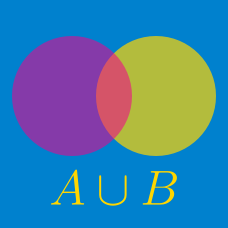Probability

# Sets - Operations on Multiple sets

Consider a ground set $U$ and let $A,B$ and $C$ be subsets of $U$ such that \begin{aligned} & \lvert U \rvert =110, \lvert A \rvert =44, \lvert B \rvert =27, \\ & \lvert C \rvert =47, \lvert A \cup B \cup C \rvert=90. \end{aligned} If no element belongs to exactly two of the three subsets, what is the number of elements in the complement of $A \cap B \cap C?$

Consider a ground set $U$ and let $X,Y$ and $Z$ be subsets of $U$ such that \begin{aligned} \lvert U \rvert & =140, \lvert X \rvert =40, \lvert Y \rvert =35, \lvert Z \rvert=53, \\ \lvert X \cap Y \rvert &=7, \lvert Y \cap Z \rvert=10, \lvert Z \cap X \rvert=15, \\ & \lvert X \cap Y \cap Z \rvert=3. \end{aligned} What is the number of elements in the complement of $X \cup Y \cup Z?$

Given a universal set $U$ and subsets $A, B$ and $C$ of $U$, which of the following is equivalent to the set $\left\{ A\cap (A^{ c }\cup B) \right\} \cup \left\{ B\cap (B\cup C) \right\}?$

$100$ students took a quiz with the three problems Easy, Medium, and Challenge. $52$ solved Easy, $35$ students solved Medium, and $32$ students solved Challenge. Also, $37$ students solved exactly two out of the three problems and $10$ students solved all three problems. How many students solved none of the three problems?

Suppose $A$, $B$ and $C$ are three sets such that $A \cap B=\emptyset, \lvert A \rvert =9, \lvert B \rvert=9,$ $\lvert C \rvert=6, \lvert A \cup C \rvert =14, \lvert B \cup C \rvert=10.$ What is the number of elements in the set $A \cup B \cup C?$

×